# Voltage

Voltage is the difference in electric potential between two points. The symbol for voltage is V or U and it is measured in volts (V), which is defined as joule per coulomb.

### Ohm's law

Ohm's law

Ohm's law describes the relation between Voltage, Current and Resistance. The law states that the voltage is equal to the current multiplied by the resistance. Ohm's law is named after the German physicist Georg Ohm.

#### Formulas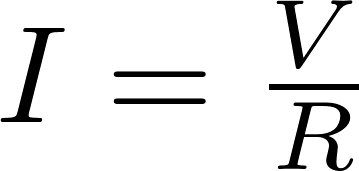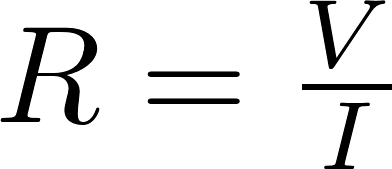V is the symbol for voltage and is measured in volt (V).
I is the symbol for current and is measured in ampere (A).
R is the symbol for resistance and is measured in ohm (Ω).

### Voltage drop

Voltage drop

The voltage drop is the voltage that is lost in a wire due to its resistance. The voltage drop is calculated with the following formula: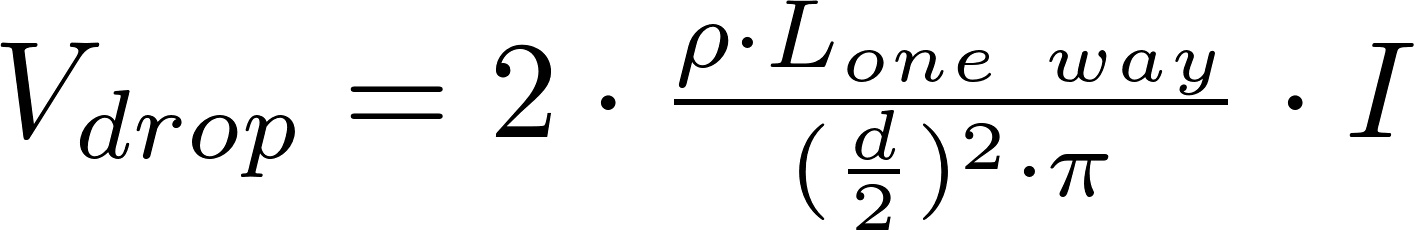### RMS voltage

RMS voltage

The RMS voltage can be calculated with one of the following formulas: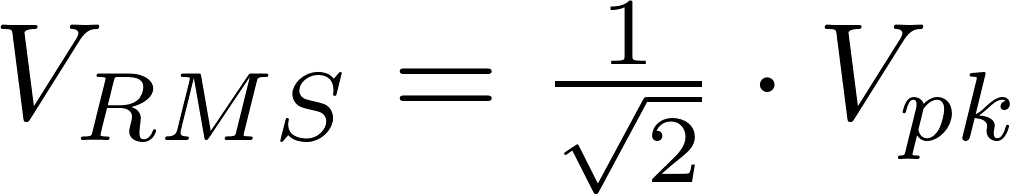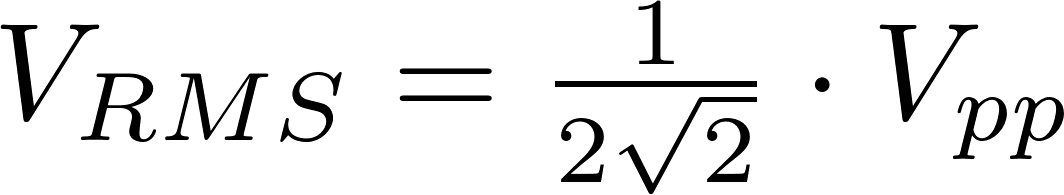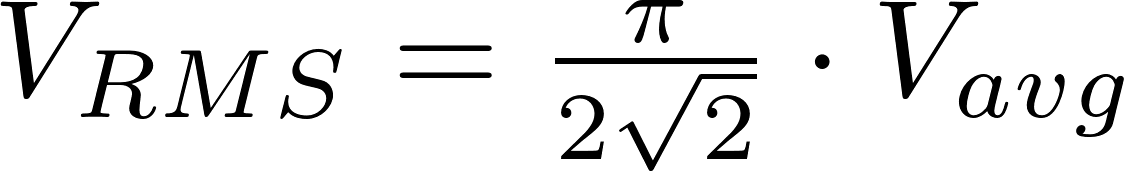V RMS is the symbol for RMS voltage and is measured in volt (V).
V pk is the symbol for peak voltage and is measured in volt (V).
V pp is the symbol for peak-to-peak voltage and is measured in volt (V).
V avg is the symbol for average voltage and is measured in volt (V).